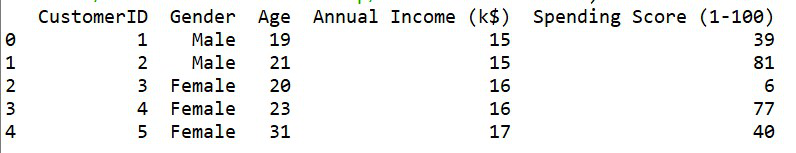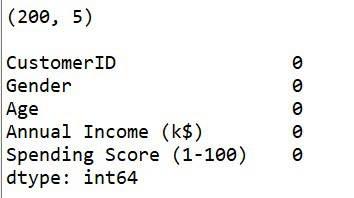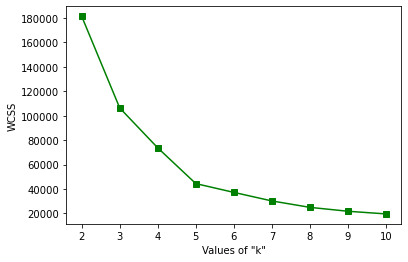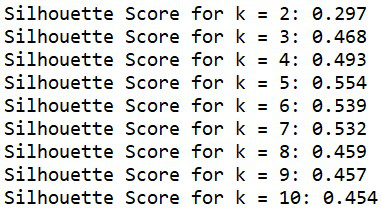GeeksforGeeks App
Open AppBrowser
Continue

# Determining the Number of Clusters in Data Mining

In Clustering algorithms like K-Means clustering, we have to determine the right number of clusters for our dataset. This ensures that the data is properly and efficiently divided. An appropriate value of ‘k’ i.e. the number of clusters helps in ensuring proper granularity of clusters and helps in maintaining a good balance between compressibility and accuracy of clusters.

Let us consider two cases:

```Case 1: Treat the entire dataset as one cluster
Case 2: Treat each data point as a cluster```

This will give the most accurate clustering because of the zero distance between the data point and its corresponding cluster center. But, this will not help in predicting new inputs. It will not enable any kind of data summarization.

So, we can conclude that it is very important to determine the ‘right’ number of clusters for any dataset. This is a challenging task but very approachable if we depend on the shape and scaling of the data distribution. A simple method to calculate the number of clusters is to set the value to about √(n/2) for a dataset of ‘n’ points. In the rest of the article, two methods have been described and implemented in Python for determining the number of clusters in data mining.

### 1. Elbow Method:

This method is based on the observation that increasing the number of clusters can help in reducing the sum of the within-cluster variance of each cluster. Having more clusters allows one to extract finer groups of data objects that are more similar to each other. For choosing the ‘right’ number of clusters, the turning point of the curve of the sum of within-cluster variances with respect to the number of clusters is used. The first turning point of the curve suggests the right value of ‘k’ for any k > 0. Let us implement the elbow method in Python.

Step 1: Importing the libraries

## Python3

 `# importing the libraries``import` `pandas as pd``import` `matplotlib.pyplot as plt``from` `sklearn.cluster ``import` `KMeans`

We have used the Mall Customer dataset which can be found on this link.

## Python3

 `# loading the dataset``dataset ``=` `pd.read_csv(``'Mall_Customers.csv'``)` `# printing first five rows of the dataset``print``(dataset.head(``5``))`

Output:First five rows of the dataset

Step 3: Checking for any null values

The dataset has 200 rows and 5 columns. It has no null values.

## Python3

 `# printing the shape of dataset``print``(dataset.shape)` `# checking for any``# null values present``print``(dataset.isnull().``sum``())`

Output:Shape of the dataset along with count of null values

Step 4: Extracting 2 columns from the dataset for clustering

Let us extract two columns namely ‘Annual Income (k\$)’ and ‘Spending Score (1-100)’ for further process.

## Python3

 `# extracting values from two``# columns for clustering``dataset_new ``=` `dataset[[``'Annual Income (k\$)'``,``                       ``'Spending Score (1-100)'``]].values`

Step 5: Determining the number of clusters using the elbow method and plotting the graph

## Python3

 `# determining the maximum number of clusters``# using the simple method``limit ``=` `int``((dataset_new.shape[``0``]``/``/``2``)``*``*``0.5``)` `# selecting optimal value of 'k'``# using elbow method` `# wcss - within cluster sum of``# squared distances``wcss ``=` `{}` `for` `k ``in` `range``(``2``,limit``+``1``):``    ``model ``=` `KMeans(n_clusters``=``k)``    ``model.fit(dataset_new)``    ``wcss[k] ``=` `model.inertia_``    ` `# plotting the wcss values``# to find out the elbow value``plt.plot(wcss.keys(), wcss.values(), ``'gs-'``)``plt.xlabel(``'Values of "k"'``)``plt.ylabel(``'WCSS'``)``plt.show()`

Output:Plot of Elbow Method

Through the above plot, we can observe that the turning point of this curve is at the value of k = 5. Therefore, we can say that the ‘right’ number of clusters for this data is 5.

### 2. Silhouette Score:

Silhouette score is used to evaluate the quality of clusters created using clustering algorithms such as K-Means in terms of how well data points are clustered with other data points that are similar to each other. This method can be used to find the optimal value of ‘k’. This score is within the range of [-1,1]. The value of ‘k’ having the silhouette score nearer to 1 can be considered as the ‘right’ number of clusters. sklearn.metrics.silhouette _score() is used to find the score in Python. Let us implement this for the same dataset used in elbow method.

Step 1: Importing libraries

## Python3

 `# importing the libraries``import` `pandas as pd``import` `numpy as np``import` `matplotlib.pyplot as plt``from` `sklearn.cluster ``import` `KMeans``from` `sklearn.metrics ``import` `silhouette_score`

We have used the Mall Customer dataset.

## Python3

 `# loading the dataset``dataset ``=` `pd.read_csv(``'Mall_Customers.csv'``)` `# printing first five rows of the dataset``print``(dataset.head(``5``))`

Output:First five rows of the dataset

Step 3: Checking for any null values

The dataset has 200 rows and 5 columns. It has no null values.

## Python3

 `# printing the shape of dataset``print``(dataset.shape)` `# checking for any``# null values present``print``(dataset.isnull().``sum``())`

Output:Shape of the dataset along with count of null values

Step 4: Extracting 2 columns from the dataset for clustering

Let us extract two columns namely ‘Annual Income (k\$)’ and ‘Spending Score (1-100)’ for further process.

## Python3

 `# extracting values from two``# columns for clustering``dataset_new ``=` `dataset[[``'Annual Income (k\$)'``,``                       ``'Spending Score (1-100)'``]].values`

Step 5: Determining the number of clusters using silhouette score

The minimum number of clusters required for calculating silhouette score is 2. So the loop starts from 2.

## Python3

 `# determining the maximum number of clusters``# using the simple method``limit ``=` `int``((dataset_new.shape[``0``]``/``/``2``)``*``*``0.5``)` `# determining number of clusters``# using silhouette score method``for` `k ``in` `range``(``2``, limit``+``1``):``    ``model ``=` `KMeans(n_clusters``=``k)``    ``model.fit(dataset_new)``    ``pred ``=` `model.predict(dataset_new)``    ``score ``=` `silhouette_score(dataset_new, pred)``    ``print``(``'Silhouette Score for k = {}: {:<.3f}'``.``format``(k, score))`Silhouette scores for k = [2,..,10]

As we can observe, the value of k = 5 has the highest value i.e. nearest to +1. So, we can say that the optimal value of ‘k’ is 5.

Now, we have rightly determined and validated the number of clusters for the Mall Customer Dataset using two methods – elbow method and silhouette score. In both the cases, k = 5. Let us now perform KMeans clustering on the dataset and plot the clusters.

## Python3

 `# clustering the data using Kmeans``# using k = 5``model ``=` `KMeans(n_clusters``=``5``)` `# predicting the clusters``pred ``=` `model.fit_predict(dataset_new)` `# plotting all the clusters``colours ``=` `[``'red'``, ``'blue'``, ``'green'``, ``'yellow'``, ``'orange'``]` `for` `i ``in` `np.unique(model.labels_):``    ``plt.scatter(dataset_new[pred``=``=``i, ``0``],``                ``dataset_new[pred``=``=``i, ``1``],``                ``c ``=` `colours[i])``    ` `# plotting the cluster centroids``plt.scatter(model.cluster_centers_[:, ``0``],``            ``model.cluster_centers_[:, ``1``],``            ``s ``=` `200``,  ``# marker size``            ``c ``=` `'black'``)` `plt.title(``'K Means clustering'``)``plt.xlabel(``'Annual Income (k\$)'``)``plt.ylabel(``'Spending Score (1-100)'``)``plt.show()`Final Clusters so formed

From the above plot, we can see that five efficient clusters have been formed which are clearly separable from each other. The cluster centroids are also visible in black color.

My Personal Notes arrow_drop_up